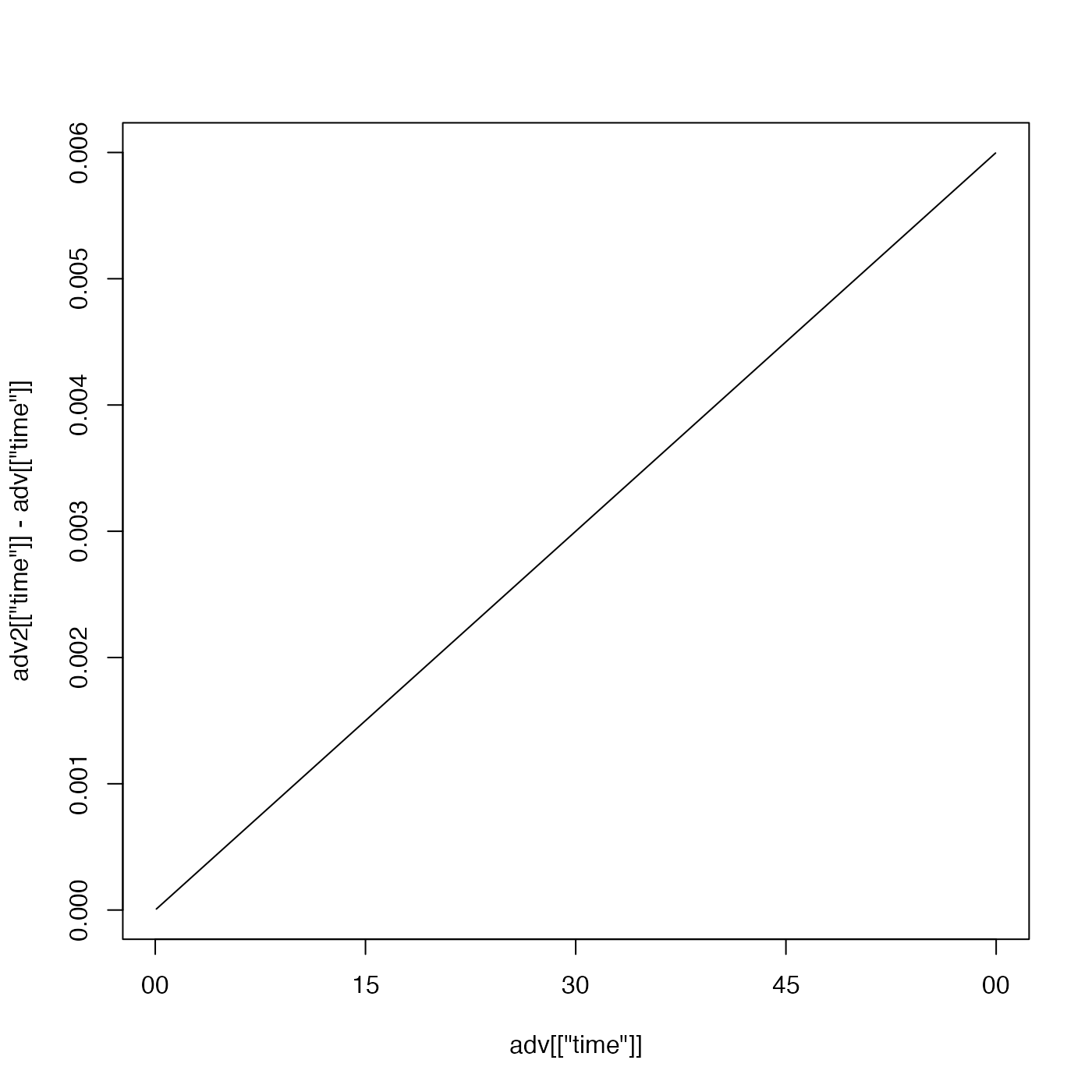This function compensates for drifting instrument clocks, according to $$t'=t + a + b (t-t0)$$, where $$t'$$ is the true time and $$t$$ is the time stored in the object. A single check on time mismatch can be described by a simple time offset, with a non-zero value of a, a zero value of b, and an arbitrary value of t0. Checking the mismatch before and after an experiment yields sufficient information to specify a linear drift, via a, b, and t0. Note that t0 is just a convenience parameter, which avoids the user having to know the "zero time" used in R and clarifies the values of the other two parameters. It makes sense for t0 to have the same timezone as the time within x.

retime(x, a, b, t0, debug = getOption("oceDebug"))

## Arguments

x an oce object. intercept (in seconds) in linear model of time drift (see “Details”). slope (unitless) in linear model of time drift (unitless) (see “Details”). reference time (in POSIXct() format) used in linear model of time drift (see “Details”). a flag that, if nonzero, turns on debugging.

## Value

A new object, with time and other data adjusted.

## Details

The returned object is computed by linear interpolation, using approx() with rule=2, to avoid NA values at the start or end. The new time will be as given by the formula above. Note that if the drift is large enough, the sampling rate will be changed. It is a good idea to start with an object that has an extended time range, so that, after this is called, subset() can be used to trim to a desired time range.

## Examples

library(oce)
plot(adv[["time"]], adv2[["time"]]-adv[["time"]], type='l')